# LCM of two number

Find the smallest multiple of 63 and 147

x =  441

### Step-by-step explanation: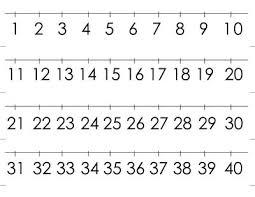Did you find an error or inaccuracy? Feel free to write us. Thank you!Tips to related online calculators
Do you want to calculate least common multiple two or more numbers?

## Related math problems and questions:

• LCMCommon multiple of three numbers is 3276. One number is in this number 63 times, second 7 times, third 9 times. What are the numbers?
• Lcm simpleFind least common multiple of this two numbers: 140 175.
• Lcm 2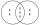Create the smallest possible number that is divisible by numbers 5,8,9,4,3
• Lcm of three numbersWhat is the Lcm of 120 15 and 5
• Find unknown numberWhat is the number between 50 and 55 that is divisible by 2,3,6,9?
• DecomposeDecompose into primes and find the smallest common multiple n of (16,20) and the largest common divisor D of the pair of numbers (140,100)
• LCMWhat is the least common multiple of 5, 50, 14?
• Counting numberWhat is the smallest counting number divisible by 2,5,7,8 and 15?
• LCD 2The least common denominator of 2/5, 1/2, and 3/4
• The smallest numberWhat is the smallest number that can be divided by both 5 and 7
• Reminder and quotientThere are given numbers A = 135, B = 315. Find the smallest natural number R greater than 1 so that the proportions R:A, R:B are with the remainder 1.
• Two hundred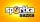Two hundred ten athletes competed in three athletics races on three fields. One hundred five athletes competed in the first, 60 in the second, and everyone else in the third. On the individual courts, the athletes were divided into groups. Each group, alt
• Game roomWinner can took win in three types of jettons with value 3, 30 and 100 dollars. What is minimal value of win payable in this values of jettons?
• RemaindersIt is given a set of numbers { 170; 244; 299; 333; 351; 391; 423; 644 }. Divide this numbers by number 66 and determine set of remainders. As result write sum of this remainders.
• Buses 4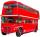intervals: 1st bus 40 min. 2nd bus 2h 3rd bud 20min How long take them to meet - as soon as possible?
• Clock's gears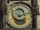In the clock machine, three gears fit together. The largest has 168 teeth, the middle 90 teeth, and the smallest 48 teeth. The middle wheel turns around its axis in 90 seconds. How many times during the day do all the gears meet in the starting position?
• The King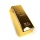The King wants to divide his sons equally. He has 42 rubies and 45 diamonds. How many sons and how will they share them?